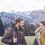Limits and their consequences

I got to this question

$\lim_{n\rightarrow \infty }e^{-n}\sum_{k=0}^{n}\frac{n^k}{k!} = \frac{1}{2}$

I asked some profs, but they handed me solutions using Poisson distribution or lots of integrals. This was supposed to be an exercise by my teacher that could be solved using some basic properties of limits (their arithmetics, squeeze theorem etc.), definition of $e^x$ as $\lim_{n \rightarrow \infty} (1+\frac{x}{n})^n$ basic limits with e, binomial expansion and logarithms, but without using integrals, series, Stirling formula, asymptotics, Taylor series?

My teacher claims it can be solved with knowledge introduced on lectures so far, which is not much, mainly things mentioned above. Of course, I can use theorems not mentioned on the lectures, but then I have to prove them, and again, with the baisc knowledge. I've been thinking about it for a few days and couldn't do any major progress in my attempts.

Any help would help :-)Note by Department 8
7 months ago

MarkdownAppears as
*italics* or _italics_ italics
**bold** or __bold__ bold
- bulleted- list
• bulleted
• list
1. numbered2. list
1. numbered
2. list
Note: you must add a full line of space before and after lists for them to show up correctly
paragraph 1paragraph 2

paragraph 1

paragraph 2

[example link](https://brilliant.org)example link
> This is a quote
This is a quote
    # I indented these lines
# 4 spaces, and now they show
# up as a code block.

print "hello world"
# I indented these lines
# 4 spaces, and now they show
# up as a code block.

print "hello world"
MathAppears as
Remember to wrap math in $ ... $ or $ ... $ to ensure proper formatting.
2 \times 3 $2 \times 3$
2^{34} $2^{34}$
a_{i-1} $a_{i-1}$
\frac{2}{3} $\frac{2}{3}$
\sqrt{2} $\sqrt{2}$
\sum_{i=1}^3 $\sum_{i=1}^3$
\sin \theta $\sin \theta$
\boxed{123} $\boxed{123}$

Sort by:

- 7 months ago

Well, I tried to look into it but the solution and the available link provided me with nothing of the fact I was looking, as we have not studied that now.

- 7 months ago